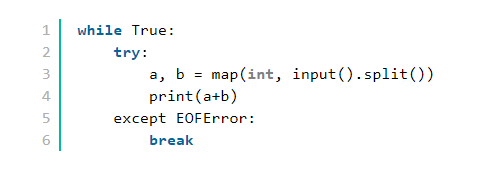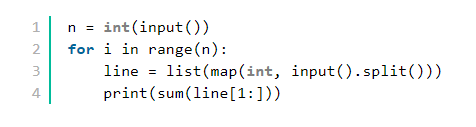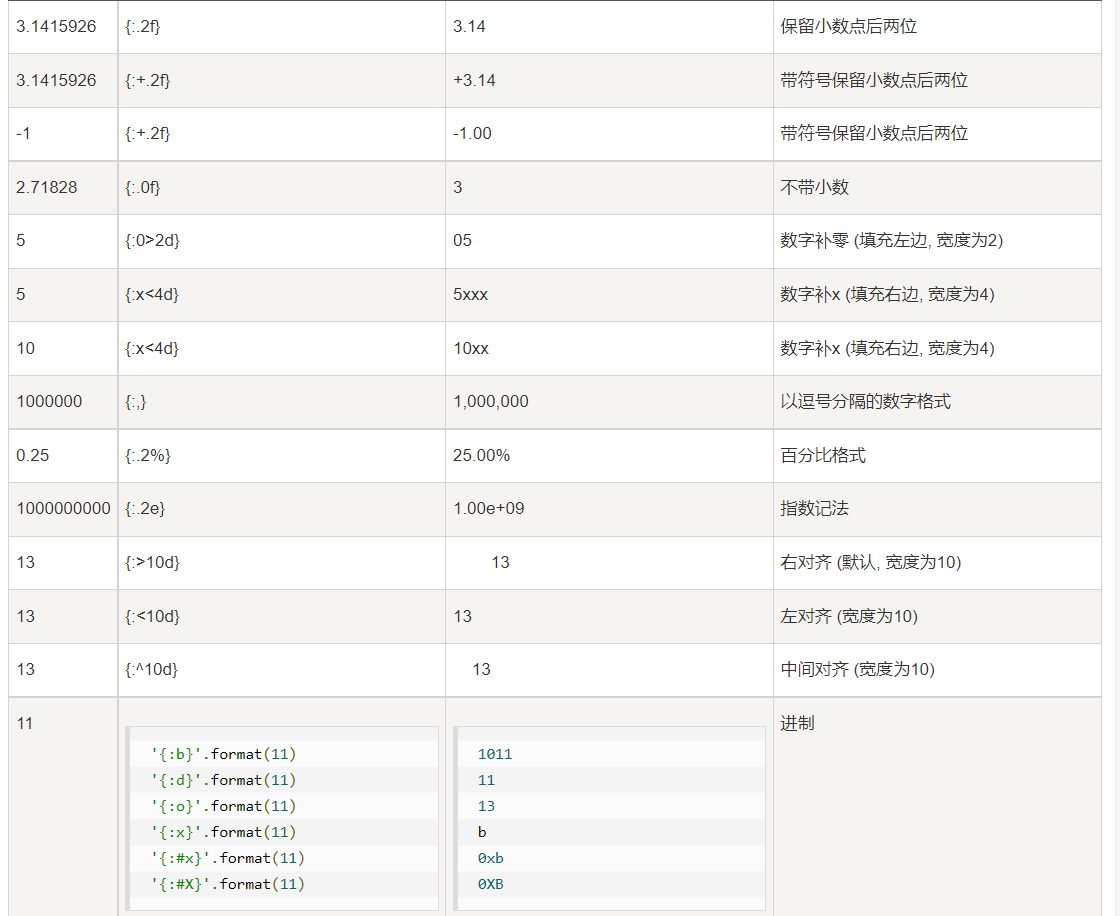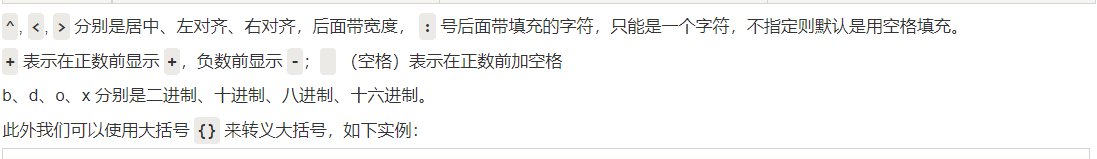## 1. 对于list排序，可以返回排序好的list，也可以返回根据list的值排序的index。

``````#对list进行排序，使用sort函数，该函数可以对list本身进行操作，所以不需要再有一个变量来获取排序的结果
lis = [1,2,3,0,1,9,8]
sort(lis)
print(lis)
>>[0,1,1,2,3,8,9]

``````
``````#需要返回list排序的索引，需要用到sorted函数。
#sorted(iterable, key=None, reverse=False),sorted有三个参数,具体含义可自行搜索。
#sorted的操作结果需要用一个变量去获取，例如下面的a
lis = [1,2,3,0,1,9,8]
a = sorted(range(len(lis)), key=lambda k: lis[k])
print(a)
>>[3, 0, 4, 1, 2, 6, 5]
#range(len(lis))返回的是一个可迭代对象，也可以写成一个数组，即
#替换为[i for i in range(len(lis))]，也就是[0,1,2,3,4,5,6]
#lambda是匿名函数，这个函数的传入参数是k，函数的执行体为lis[k]
#key值就是排序的依据，也就是把需要排序的lis遍历一遍
``````

sorted还有很多便捷的操作，如果有多个指标，可以指定某一个指标进行排序，具体的用法可以参考这里：sorted用法

## 2. Python获取输入的数据流

``````#获取输入数据流，以换行符为结束标志，换行符之前的所有输入都会被记录在一个变量中，例如输入的是2，3，4
k = input()
>> k = "2,3,4"
``````

input函数接收到的内容都被转换为str类型，规则性输入的时候可以直接用split函数转换成列表方便后续应用。

``````#如果输入是规则的，即都用逗号或空格分割，可以使用split函数。
#例如输入的是2，3，4
k = input().split(",")
>> k = ['2','3','4']
#结合map函数，使list的类型强制转换
k = list(map(int,k))
>>k=[2,3,4]
``````## 3. 创建一个list

``````listA = []
``````

``````#返回一个长度为15的list，且每个值都为0
listB =  * 15
``````

``````#返回0-9的递增list
listC = [i for i in range(10)]
``````

## 4. list操作

``````a = [1,2,3]
b = a[::-1]
print(b)
>>[3,2,1]
``````

list的倒序索引:-1是最后一个，其他依次往前递减

``````a = [1,2,3,4,5]
b = a[-3:-1]
print(b)
>> [3,4]
print(a[-1])
>>5
print(a[-3])
>>3
``````

list的切片索引：

``````a = [1,2,3,4,5,6,7]
b = a[1:6:2]#从下标为1开始算起，到3为止，步长为2取值。
>>[2, 4, 6]
c = a[1:6:3]
>>[2, 5]
``````

list的无限索引：[::step],step表示步长

``````a = [1,2,3,4,5]
b = a[::3] #如果step为正数，则和切片索引一样。
>> b = [1,4]
c = a[::-3]#如果step为负数，也是和切片索引一样取值，但是是从后往前取值，且最后一个的下标为-1.
>> c = [5,2]
d = a[::8]#如果step超出list的长度，则只返回第一个值
>> d = 1
e = a[::-8]
>> e = 5
``````

``````#按索引删除
del a
print(a)
>>[2,3]
#按值删除
a.remove(3)
print(a)
>>
``````

pop函数，弹出一个值，并删除这个值

``````#pop的index默认为0，-1表示最后一个
a = [1,2,3]
b = pop(index = 0)
>> a =[2,3]
>>> b = 1
``````

clear操作，情况list

``````a = [1,2,3]
a.clear()
>>a=[]
``````

list相加

``````a = [1,2,3]
a = a + [4,5]
>> a = [1,2,3,4,5]

b = [6,7]
a += b
>> a= [1,2,3,4,5,6,7]

a += [c]
``````

## 5. 矩阵90度旋转

``````#矩阵逆时针旋转90°
def trans90(self,matrix):
res = []
for line in zip(*matrix):
res.append(list(line))
return res[::-1]
``````

## 6. 二进制

``````def NumberOf1(self, n):
# write code here
# 直接进行位操作，与16进制的 8个f，即32个1 进行与操作。
#负数时，会直接得到该负数的补码
#正数时，原码与补码相同，得到的结果
n = n & 0xffffffff
str = bin(n)[2:]
return str.count('1')
``````

``````a = bin(8)
>> a = 0b1000
b = bin(-8)
>>b=-0b1000
``````

## 7. list类型转换 一维/二维

``````#一维list类型转换
tt=['1','2','3']
res = list(map(int,tt))
>>res = [1,2,3]
``````
``````#二维list类型转换，本质上还是以为类型转换的方法
tt = [['1','2','3'],['4','5','6'],['7','8','9']]
res = []
for i in range(len(tt)):
temp = list(map(int,tt[i]))
res.append(temp)
>> res = [[1, 2, 3], [4, 5, 6], [7, 8, 9]]
#或者可以把for循环简写成如下格式
[res.append(list(map(int,tt[i]))) for i in range(len(tt))]
``````

## 8. 拼接 : " ".join()

``````#用空格拼接
a = ['this','is','an','apple']
b = " ".join(a)
>>b = this is an apple
#如果不需要有空格，则是
c = "".join(a)
>> c = thisisanapple
#join函数中必须是str类型，如果不是需要强制类型转换
d = [1,2,3]
e = "".join([str(i) for i in d])
>> d = 123
``````

## 9. list降维

``````cc=[[1,2,3,4]]
# for i in cc:
# 	a = i
print(a)
>> a = [1,2,3,4]
#或者采用for循环的简写形式，
a = [j for i in cc for j in i]
print(a)
>> a = [1,2,3,4]
``````

``````cc = [[1, 2, 3], [4, 5, 6], [7, 8, 9]]
a = []
for i in cc:
a += i
>> a = [1,2,3,4,5,6,7,8,9]
#或者采用和第一种形式相同的写法
a = [j for i in res for j in i]
>> a = [1,2,3,4,5,6,7,8,9]
``````

## 10. for的简写

``````for i in range(n):
对i的操作
#可简写成
[ 对i的操作 for i in 列表 ]
``````

``````#[对i的操作 for 单个元素 in 列表 for i in 单个元素]
y_list = [[1,2,3],[4,5,6]]
[print(i) for y in y_list for i in y]

>>1 2 3 4 5 6
``````

## 11. if的简写

``````True的逻辑 if 条件 else False的逻辑
``````

``````y = 0
x = y+3 if y > 3 else y-1
>> x=-1
``````

for循环和if判断相结合的缩写形式：

``````[判断为True的i的操作 for i in 列表 if i的判断 ]
``````

``````x = [1,2,3,4,5,6,7]
[print(i) for i in x if i > 3 ]
>> 4,5,6,7
``````

## 12. 匿名函数lambda

``````lambda k: 2*k
``````

``````a = 3
lambda k: 2*k（a）
>> 6
``````

## 13 按条件筛选list的值-filter函数

``````arr =[8,-1,3,9,5]
a = list(filter(lambda x:x>0, arr))
>> a = [8,3,9,5]
``````

list的index函数，如果找到则返回下标，如果找不到，则抛出异常。

``````a = [1,2,3]
try:
i = a.index(4)
print(i)
except:
print("没找到！")
>>没找到！
``````

## 14. 位操作

& : 按位与操作, 只有 1&1 为 1, 其它情况为 0. 可用于进位运算
| : 按位或操作 , 只有 0|0 为0 ,其他情况为1
~ : 逐位取反
^ : 异或, 相同为 0 ,相异为 1 . 可用于加操作 (不包括进位项 )
<< : 左移操作, 2的幂有关,×2
’ >>:右移操作, 2的幂有关,÷2

`````` 异或应用：给定一个非空整数数组，除了某个元素只出现一次以外，其余每个元素均出现两次。找出那个只出现了一次的元素。
#异或同一个数字两次，则原数字不变
a = [1,2,3,4,3,2,1]
res = 0
for i in a:
res ^= i
print(res)
>>res = 4
``````
``````左移操作,右移操作
a = 2
a = a<<1 #左移一位，相当于×2
>> a = 4
a = a<<2 #左移两位，相当于×4（2的2次）
>> a = 16
b = a>>1 #右移1位，相当于÷2
>>b = 8
b = b >>2 #右移两位，相当于÷4（2的2次）
>>b = 2
``````

## 15. python的内置函数总结解析

• abs函数，求绝对值。
• all()函数，用于判断所有元素是否都为 TRUE，如果包含 0、空、None、False，则返回False，否则返回True
• any()函数，如果元组或列表都为空、0、false，则返回false，如果不都为空、0、false，则返回true。
• basestring()函数是 str 和 unicode 的超类（父类），也是抽象类，因此不能被调用和实例化，但可以被用来判断一个对象是否为 str 或者 unicode 的实例，isinstance(obj, basestring) 等价于 isinstance(obj, (str, unicode))。Python3 不支持 basestring() 函数，改用 str() 函数
``````>>>isinstance("Hello world", str)
True
>>> isinstance("Hello world", basestring)
True
``````
• bin() 返回一个整数 int 或者长整数 long int 的二进制表示。
``````>>>print(bin(10))
>0b1010
>>>print(bin(-10))
>-0b1010
``````
• bool()函数，进行类型转换，0是False，其余为True
``````>>>bool()
False
>>> bool(0)
False
>>> bool(1)
True
>>> issubclass(bool, int)  # bool 是 int 子类
True
``````
• chr()函数 用一个范围在 range（256）内的（就是0～255）整数作参数，返回一个对应的字符。
``````#可以使用该函数实现数字到字母的转化，A的ASCII为65
>>>print(chr(1+65)
B
``````
• ord() 函数是 chr() 函数的配对函数，它以一个字符作为参数，返回对应的 ASCII 数值，或者 Unicode 数值，如果所给的 Unicode 字符超出了你的 Python 定义范围，则会引发一个 TypeError 的异常。
``````>>>print(ord('B'))
66
>>>print(ord('B')-ord('A'))
1
``````
• cmp(x,y) 函数用于比较2个对象，如果 x < y 返回 -1, 如果 x == y 返回 0, 如果 x > y 返回 1.
python3.x中已经没有cmp函数，如果你需要实现比较功能，需要引入 operator 模块。
• complex() 函数用于创建一个值为 real + imag * j 的复数或者转化一个字符串或数为复数。如果第一个参数为字符串，则不需要指定第二个参数。
``````>>>print(complex(1,2))
1+2j
``````
• dict()函数 用于创建一个字典。
``````>>> d = dict()
{
}
>>> d = {
}
{
}
``````
• int(),list(),float(),long(),str()都是类型转换的函数
• set() 函数创建一个无序不重复元素集，可进行关系测试，删除重复数据，还可以计算交集、差集、并集等。
• frozenset() 函数返回一个冻结的集合，冻结后集合不能再添加或删除任何元素。
• tuple()函数将列表转换为元组。
• divmod() 函数把除数和余数运算结果结合起来，返回一个包含商和余数的元组(a // b, a % b)
``````>>>divmod(3,2)
(1,1)
``````
• eval() 函数用来执行一个字符串表达式，并返回表达式的值。
``````>>>x = 7
>>> eval( '3 * x' )
21
>>> eval('pow(2,2)')
4
``````
• enumerate() 函数用于将一个可遍历的数据对象(如列表、元组或字符串)组合为一个索引序列，同时列出数据和数据下标，一般用在 for 循环当中。
``````>>>a = [i for i in range(9,6,-1)]
[9,8,7]
>>>for index,value in enumerate(a):
print(index,value)
0 9
1 8
2 7
``````
• filter() 函数用于过滤序列，过滤掉不符合条件的元素，返回由符合条件元素组成的新列表。Python3中filter函数返回的是一个filter类，需要用list()转换为list类型。
``````filter(function, iterable)
function -- 判断函数。
iterable -- 可迭代对象。
>>>a = [1,2,3,4]
res = list(filter(lambda x:x%2,a))
print(res)
[1,3]
``````
• format()函数用于格式化
``````字符串的格式化
>>>s = ["Hello","world" ]
c = "{0} {1} {0}".format(s,s)
print(c)
Hello world Hello
``````
``````数字格式化
>>> print("{:.2f}".format(3.1415926))
3.14
>>> print("{:+.2f}".format(3.1415926))
+3.14
>>> print("{:+.2f}".format(-1))
-1.00
``````• round() 方法返回浮点数x的四舍五入值。
``````round( x [, n]  )
>>>print(round(3.14159))
3
>>>print(round(3.14159,2))
3.14
``````
• id() 函数返回对象的唯一标识符，也就是对象在内存中的地址。
``````>>>a = 3
print(id(a))
140736474878784
>>>a = 1
print(id(a))
140736474878784
``````
``````>>>a = [1,2,3,4]
print(id(a))
#list的id多次输出结果不一致
2553140961928
2242628444808
1718718194312
2274422121096
>>>print(id(a))
#多次输出结果一致
140736436933440
140736436933440
``````
• hash()函数 用于获取取一个对象（字符串或者数值等）的哈希值。
• help()函数用于查看函数或模块用途的详细说明。
``````help('str')
help('list.append')
>>>a = [1,2,3]
help('a.pop')
``````
• hex() 函数用于将10进制整数转换成16进制，以字符串形式表示。
• oct() 函数将一个整数转换成 8 进制字符串，Python3.x 版本的 8 进制以 ‘0o’ 作为前缀表示。
• int()函数也除了可以类型转换，也可用于进制转换
``````>>>print(int('111',2))
7
>>>print(int('111',8))
73
>>>print(int('111',16))
273
>>>print(hex(273))
0x111
>>>print(hex(-273))
-0x111
``````
• isinstance() 函数来判断一个对象是否是一个已知的类型，类似 type()。
``````>>>a = 2
>>> isinstance (a,int)
True
>>> isinstance (a,str)
False
>>> isinstance (a,(str,int,list))    # 是元组中的一个返回 True
True
``````
• type() 函数如果你只有第一个参数则返回对象的类型，三个参数返回新的类型对象。
• max() 方法返回给定参数的最大值，参数可以为序列。
• min() 方法返回给定参数的最小值，参数可以为序列。
max和min函数不仅可以对数字操作，也可以选择str的最大或最小值
``````>>>s = ['aa','bb','abc','da']
print(max(s))
da
>>>print(min(s))
aa
>>>s.sort()
print(s)
['aa', 'abc', 'bb', 'da']

``````
• next()函数 返回迭代器的下一个项目。
next() 函数要和生成迭代器的 iter() 函数一起使用。
``````# 首先获得Iterator对象:
it = iter([1, 2, 3, 4, 5])
# 循环:
while True:
try:
# 获得下一个值:
x = next(it)
print(x)
except StopIteration:
# 遇到StopIteration就退出循环
break
``````
• pow() 方法返回 （x 的 y 次方） 的值。
函数是计算 x 的 y 次方，如果 z 在存在，则再对结果进行取模，其结果等效于 pow(x,y) %z。
``````pow(x, y[, z])
``````
• sum() 方法对序列进行求和计算。
• sort()函数和sorted()函数见第一小节的内容
• reverse() 函数用于反向列表中元素。
``````>>>s = ['aa','bb','abc','da']
s.reverse()
print(s)
['da', 'abc', 'bb', 'aa']
``````
• range()函数返回的是一个可迭代对象（类型是对象），而不是列表类型， 所以打印的时候不会打印列表。list() 函数是对象迭代器，可以把range()返回的可迭代对象转为一个列表，返回的变量类型为列表。
• xrange() 函数用法与 range 完全相同，所不同的是生成的不是一个数组，而是一个生成器。
• slice() 函数实现切片对象，主要用在切片操作函数里的参数传递。
``````>>>a = [1,2,3,4,5,6,7,8]
myslice = slice(5)
print(a[myslice])
[1,2,3,4,5]
>>>myslice = slice(2,5)
print(a[myslice])
[3,4,5]
``````

（2021/10/05更新）

### Spring Boot 导出excel表格_JurenXxt的博客-程序员宅基地_springboot导出excel

Spring boot 导出Excel表格前言如何使用Spring Boot 导出excel表格，使用easyexcel提示：以下是本篇文章正文内容，下面案例可供参考一、pandas是什么？示例：pandas 是基于NumPy 的一种工具，该工具是为了解决数据分析任务而创建的。二、使用步骤1.添加POM依赖代码如下： &lt;dependency&gt; &lt;groupId&gt;com.alibaba&lt;/groupId&gt;

### spring xml读取properties.xml 文件_Gao_N的博客-程序员宅基地_xml读取properties

在配置ssm框架时，配置文件applicationContext.xml配置DataSource，读取资源文件jdbc.properties失败。刚开始我是这么写的&amp;lt;context:property-placeholder location=&quot;classpath:jdbc.properties&quot; /&amp;gt;后来修改为        &amp;lt;bean id=&quot;propertyC...

### 0-1背包问题求解归纳（动态规划法，贪心算法，回溯法，分治法和分支界限法）__更新到完整_pro_To_Life的博客-程序员宅基地

0-1背包问题是一个经典的算法问题，问题定义如下：   有n个物品   重量分别为W={w1, w1, w3, ..., wn}，   价值分别为V={v1, v2, v3, ..., vn}。   现在要将这N个物品放入允许的最大重量为w的包中，问怎样选择物品能使包中的物品总价值最大。下面分别用动态规划法，贪心算法，回溯法，分治法和分支界限法求解1.动态规划法

### is_base_of_linuxheik的博客-程序员宅基地

`is_base_of'如何工作？(How does `is_base_of` work?) C/C++IT屋 2016/10/11 20:27:02 百度翻译此文   有道翻译此文问 题How does the following code work?typedef char (&yes);typ

### jquery ajax 设置header踩得坑_小码蚁啊的博客-程序员宅基地

\$(&quot;#test&quot;).click(function() { \$.ajax({ type: &quot;GET&quot;, url: &quot;url&quot;, beforeSend: function(request) { request.setRequestHeader(&quot;token&quot;,&quot;******&quot;); }, ...